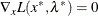### Termination Criteria

All optimization techniques stop iterating at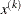if at least one of a set of termination criteria is satisfied. PROC NLP also terminates if the pointis fully constrained bylinearly independent active linear or boundary constraints, and all Lagrange multiplier estimates of active inequality constraints are greater than a small negative tolerance.

Since the Nelder-Mead simplex algorithm does not use derivatives, no termination criterion is available based on the gradient of the objective function. Powell’s COBYLA algorithm uses only one more termination criterion. COBYLA is a trust region algorithm that sequentially reduces the radius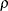of a spherical trust region from a start radius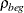= INSTEP to the final radius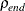= ABSXTOL. The default value is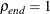e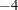. The convergence to small values of(high precision) may take many calls of the function and constraint modules and may result in numerical problems.

In some applications, the small default value of the ABSGCONV= criterion is too difficult to satisfy for some of the optimization techniques. This occurs most often when finite-difference approximations of derivatives are used.

The default setting for the GCONV= option sometimes leads to early termination far from the location of the optimum. This is especially true for the special form of this criterion used in the CONGRA optimization.

The QUANEW algorithm for nonlinearly constrained optimization does not monotonically reduce the value of either the objective function or some kind of merit function which combines objective and constraint functions. Furthermore, the algorithm uses the watchdog technique with backtracking (Chamberlain et al. 1982). Therefore, no termination criteria were implemented that are based on the values (or
) of successive iterations. In addition to the criteria used by all optimization techniques, three more termination criteria are currently available. They are based on satisfying the Karush-Kuhn-Tucker conditions, which require that the gradient of the Lagrange function is zero at the optimal point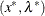: